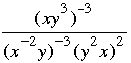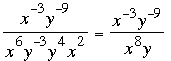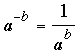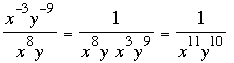SEARCH HOMEMath Central Quandaries & QueriesQuestion from Gabriel, a student: What does the line separating the top from the bottom mean? what does it tell you to do and what is the answer to the question? Question 3 Simplify. Write the answer using only positive exponents. Assume all variables represent nonzero numbers. (x^-5)^-4(x^-1y)^3 ________________ (xy^4)^3Hi Gabriel,

I am going to solve a similar problem.

Simplify. Write the answer using only positive exponents. Assume all variables represent nonzero numbers.I would first remove the parentheses remembering the two laws of exponents that say

(ab)c = abc and abac = abc.

I getWe are not done yet as there are negative exponents. Now I need to use the fact thatHence I getI hope this helps,

PennyMath Central is supported by the University of Regina and The Pacific Institute for the Mathematical Sciences.